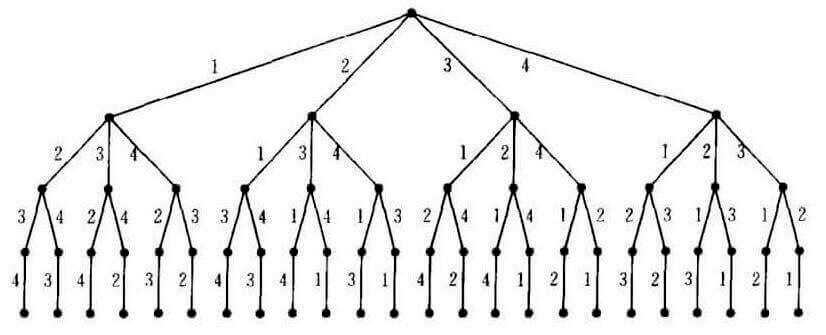# LeetCode 69. Sqrt(x)

## Description

Sqrt(x)

Implement `int sqrt(int x)`.

Compute and return the square root of x, where x is guaranteed to be a non-negative integer.

Since the return type is an integer, the decimal digits are truncated and only the integer part of the result is returned.

Example 1:

``````Input: 4
Output: 2
``````

Example 2:

``````Input: 8
Output: 2
Explanation: The square root of 8 is 2.82842..., and since
the decimal part is truncated, 2 is returned.
``````

# LeetCode 66. Plus One & 67. Add Binary

## Plus One

### Description

Plus One

Example 1:

``````Input: [1,2,3]
Output: [1,2,4]
Explanation: The array represents the integer 123.
``````

Example 2:

``````Input: [4,3,2,1]
Output: [4,3,2,2]
Explanation: The array represents the integer 4321.
``````

# LeetCode 50. Pow(x, n)

## Description

Pow(x, n)

Implement pow(x, n), which calculates x raised to the power n (x^n).

Example 1:

``````Input: 2.00000, 10
Output: 1024.00000
``````

Example 2:

``````Input: 2.10000, 3
Output: 9.26100
``````

Example 3:

``````Input: 2.00000, -2
Output: 0.25000
Explanation: 2^{-2} = {1/2}^2 = 1/4 = 0.25
``````

Note:

• -100.0 < x < 100.0
• n is a 32-bit signed integer, within the range [−2^31, 2^31 − 1]

`x``n`次方。

# LeetCode 60. Permutation Sequence

## Description

Permutation Sequence

The set `[1,2,3,...,n]` contains a total of n! unique permutations.

By listing and labeling all of the permutations in order, we get the following sequence for n = 3:

1. `"123"`
2. `"132"`
3. `"213"`
4. `"231"`
5. `"312"`
6. `"321"`

Given `n` and `k`, return the `k-th` permutation sequence.

Note:

• Given n will be between 1 and 9 inclusive.
• Given k will be between 1 and n! inclusive.

Example 1:

``````Input: n = 3, k = 3
Output: "213"
``````

Example 2:

``````Input: n = 4, k = 9
Output: "2314"
``````# LeetCode 43. Multiply Strings

Multiply Strings

## Description

Given two non-negative integers `num1` and `num2` represented as strings, return the product of `num1` and `num2`, also represented as a string.

Example 1:

Input: num1 = “2”, num2 = “3” Output: “6”

Example 2:

Input: num1 = “123”, num2 = “456” Output: “56088”

# LeetCode 29. Divide Two Integers

Divide Two Integers

## Description

Divide two integers without using multiplication, division and mod operator.

If it is overflow, return MAX_INT.Programer

ChengDu·China
-